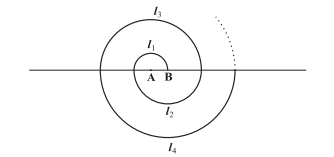# A spiral is made up of successive semicircles, with centres alternately at A and B, starting with centre at A of radii 0.5, 1.0 cm, 1.5 cm, 2.0 cm, ……… as shown in figure. What is the total length of such a spiral made up of thirteen consecutive semicircles? (Take π = 22/7)We have to find out the total length of such a spiral made up of thirteen consecutive semicircle.

Solution

We have learnt that

The perimeter of a semi-circle = πr

Hence,

P1 = π(0.5) = π/2 cm

P2 = π(1) = π cm

P3 = π(1.5) = 3π/2 cm

Where, P1, P2, P3 are the lengths of the semi-circles.

Hence we get the series as

π/2, π, 3π/2, 2π, ….

P1 = π/2 cm

P2 = π cm

Common difference, d = P– P1 = π – π/2 = π/2

First term = P1= a = π/2 cm

We know that by sum of n term formula,

Sn = n/2 [2a + (n – 1)d]

Thereforee, Sum of the length of 13 consecutive circles is;

S13 = 13/2 [2(π/2) + (13 – 1)π/2]

= 13/2 [π + 6π]

=13/2 (7π)

= 13/2 × 7 × 22/7

= 143 cm

The total length of such a spiral made up of thirteen consecutive semicircles= 143cm